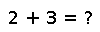# intersect

Construct the intersection of two or more sets. Maintains order and multiplicity of the first argument for arrays and ranges.

## Examples

``````julia> set1 = Set(3, 4, 5, 6)
set2 = Set(1, 3, 4)
intersect(set1, set2)
Set{Int64}({4,3})``````
``````julia> set1 = Set(3, 4, 5, 6)
set2 = Set(1, 2)
intersect(set1, set2)
Set{Int64}({})``````
``````julia> a = Set([0:3:30]);   # table of 3

julia> b = Set([0:5:50]);   # table of 5

julia> intersect(a, b)
Set{Int64}({0,15,30})``````

Checking you are not a robot: